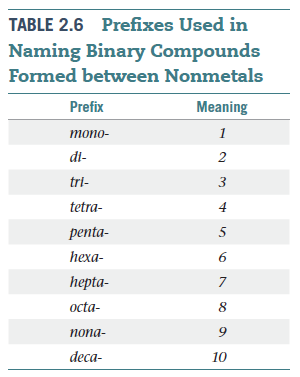Problem: Chlorine reacts with oxygen to form Cl2O7 (l).If the oxygen in Cl2O7 (l) is considered to have the -2 oxidation state, what is the oxidation state of the chlorine?

FREE Expert Solution

We’re asked to determine the oxidation state of chlorine in Cl2O7 (l), if oxygen, O is considered to have the -2 oxidation state.

Recall that the rules for oxidation states are as follows:

A. General Rules:

1. For an atom in its elemental form (Zn, Cl2, C(graphite), etc.)    O.S. = 0

2. For an ion (Li+, Al3+, etc.)                                                       O.S. = charge

B. Specific Rules:

1. Group 1A              O.S. = +1

2. Group 2A              O.S. = +2

3. Hydrogen              O.S. = +1, with nonmetals

O.S. = –1 with metals and boron

4. Fluorine                 O.S. = –1

5. Oxygen                  O.S. = –1 in peroxides (X2O2, X = Group 1A)

O.S. = –1/2 in superoxides (XO2, X = Group 1A)

O.S. = –2 in other compounds

6. Group 7A               O.S. = –1 (except when bonded to O)

Cl2O7 is a neutral covalent compound.

**Covalent compounds are formed between 2 or more nonmetals (Cl and O are nonmetals)

99% (349 ratings)
Problem Details
Chlorine reacts with oxygen to form Cl2O7 (l).If the oxygen in Cl2O7 (l) is considered to have the -2 oxidation state, what is the oxidation state of the chlorine?